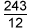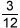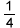# Activities

••• ##### Subject Area

• Math: Elementary Math: Fractions

• ##### AuthorK-5

30 Minutes

• ##### Device
•TI-Nspire™ CX/CX II
•TI-Nspire™ CX CAS/CX II CAS
• ##### Software

TI-Nspire™
TI-Nspire™ CAS

## Mixed Numbers

#### Activity Overview

This activity uses interactive number lines to help students investigate mixed numbers as a way to write improper fractions. Division of a whole number by a smaller whole number results in an improper fraction that can be written as a mixed number. Mixed numbers can be used to find the magnitude of an improper fraction, and division can help find the integer part of the number.

This TI-Nspire™ lesson uses interactive number lines to help students investigate mixed numbers as a way to write improper fractions. Division of a whole number by a smaller whole number results in an improper fraction that can be written as a mixed number. Mixed numbers can be used to find the magnitude of an improper fraction, and division can help find the integer part of the number (i.e.,can be thought of as 243 divided by 12, which is 20 and a remainder of 3, or 20 +or).